# Magnetic-free non-reciprocity and isolation based on parametrically modulated coupled-resonator loops

## Abstract

Non-reciprocal components, which are essential to many modern communication systems, are almost exclusively based on magneto-optical materials, severely limiting their applicability. A practical and inexpensive route to magnetic-free non-reciprocity could revolutionize radio-frequency and nanophotonic communication networks. Angular-momentum biasing was recently proposed as a means of realizing isolation for sound waves travelling in a rotating medium1, and envisaged as a path towards compact, linear integrated non-reciprocal electromagnetic components2,3. Inspired by this concept, here we demonstrate a subwavelength, linear radio-frequency non-reciprocal circulator free from magnetic materials and bias. The scheme is based on the parametric modulation of three identical, strongly and symmetrically coupled resonators. Their resonant frequencies are modulated by external signals with the same amplitude and a relative phase difference of 120°, imparting an effective electronic angular momentum to the system. We observe giant non-reciprocity, with up to six orders of magnitude difference in transmission for opposite directions. Furthermore, the device topology is tunable in real time, and can be directly embedded in a conventional integrated circuit.

## Main

Early attempts to realize magnetic-free non-reciprocity were based on the non-reciprocal properties of transistors at microwave frequencies4, and on networks of electro-optical modulators at optical frequencies5,6,7,8. However, such approaches traded the absence of magnetic bias with other significant drawbacks, such as the strong nonlinearities and poor noise-performance of transistors, or the large size and complexity of the required electro-optical networks. More recently, non-reciprocity has been achieved in transistor-loaded metamaterials9,10 and nonlinear devices11,12,13,14. Also these solutions impose severe restrictions on the input power levels, generally degrading the signal quality because of noise or signal distortion. Another interesting approach to magnetic-free non-reciprocity has been introduced15, using asymmetric mode conversion in spatiotemporally modulated waveguides. This concept is especially attractive for integrated optical networks, as it may be fully realized in silicon photonics16. However, this technique and its variants17,18,19,20,21,22 lead to structures much larger than the wavelength, owing to the weak electro-optic or acousto-optic effects on which they rely, and require complex modulation schemes. In a similar context, the concept of a non-reciprocal device based on parametrically coupled resonators has been theoretically explored23.

An approach that can lead to compact, magnetic-free non-reciprocal devices with relaxed implementation complexity was recently introduced 1,2,3, based on angular-momentum biasing of a resonant ring. Angular momentum can be applied either by mechanically spinning a fluid, as proved for acoustic waves1, or, more conveniently for electromagnetic waves, by spatiotemporal modulation with a travelling wave, realizing an effective electronic spin2,3, as illustrated in Fig. 1a. The resonant nature of the modulated ring can substantially boost the otherwise weak electro-optic effects through which spatiotemporal modulation is typically achieved24,25, allowing the design of largely non-reciprocal devices with dimensions of the order of, or even smaller than the wavelength. Furthermore, in contrast to other approaches based on spatiotemporal modulation15,19, angular-momentum biasing is based on uniform modulation across the ring cross-section, thus significantly simplifying the fabrication process.

Inspired by these ideas, here we propose a device that provides strong magnetic-free non-reciprocity at the subwavelength scale by dynamically modulating three identical resonant circuit tanks arranged in a loop and strongly coupled to each other, as in Fig. 1b. The resonant frequencies of the individual tanks are temporally modulated in a circularly rotating fashion as ω1(t) = ω0 + δωm cos(ωmt), ω2(t) = ω0 + δωm cos(ωmt + 2π/3) and ω3(t) = ω0 + δωm cos(ωmt + 4π/3), where ω0 is the static value of the resonant frequency, δωm is the modulation amplitude and ωm is the modulation frequency, so that an effective electronic spin is imparted to the system. Without modulation, the loop supports two degenerate counter-rotating modes, similar to the uniform ring of Fig. 1a. However, when the modulation is switched on, this degeneracy is lifted and non-reciprocity is induced. As we show in the following, this solution markedly boosts the modulation efficiency of the device compared to the case of a single modulated resonant ring, as considered in refs 2, 3, largely relaxing the requirements in terms of the modulation intensity and subsequently improving the overall efficiency of the structure.

In the absence of modulation (δωm = 0), the loop of Fig. 1b supports three resonant states: a common state with state vector |c〉 = [1 1 1]T and frequency ωc = ω0 + 2κ, and two degenerate right- and left-handed states with state vectors |±〉 = [1 e±i2π/3 e±i4π/3]T and frequencies ω± = ω0κ, where κ is the coupling coefficient. The applied modulation mixes right- and left-handed states, producing two new hybrid states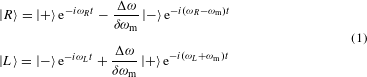where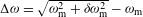, ωR = ω± − Δω/2 and ωL = ω± + Δω/2, as analytically derived in the Methods for ωm ωcω±.

It is interesting to observe that both |R〉 and |L〉 consist of a dominant state at ωR, L and a secondary state, red- or blueshifted by ωm, as in the case of the uniformly modulated ring of refs 2, 3. However, despite this apparent resemblance in the form of |R〉 and |L〉, the mechanism that creates the frequency separation Δω between resonant states in the geometry analysed here is significantly different from the uniformly modulated ring of refs 2, 3. For a uniform resonant ring (Fig. 1a), Δω is the result of an azimuthally travelling wave modulation Δɛm cos(ωmtlmϕ), where Δɛm and lm are the modulation amplitude and azimuthal order, respectively. If lm = 2l, where l is the resonant order of the ring, δωm = ωlΔɛm/(2ɛ) (refs 2, 3), where ωl is the resonant frequency and ɛ the static permittivity. The ideal continuous modulation assumed in Fig. 1a (top) is difficult, if not impossible, to realize, and an angular discretization is typically required. A discrete modulation profile with N different modulation regions, as sketched in Fig. 1a (bottom), is equivalent to a continuous effective modulation with reduced effective amplitude Δɛm, eff = Δɛmsinc(2l/N) (ref. 3), revealing a fundamental trade-off between fabrication complexity, proportional to N, and non-reciprocal response. As an example, for N = 3—that is, the minimum value for which Δɛm, eff ≠ 0—and l = 50—a typical value in realistic high-Q micro-ring resonators at 1.55 μm—Δɛm, eff = 0.008Δɛm, implying that only 0.8% of the modulation signal is effectively used to generate non-reciprocity.

In contrast, in the composite resonant loop of Fig. 1b the frequency splitting is achieved by modulating the frequency of each resonator, which can be obtained by applying a uniform permittivity modulation with amplitude Δɛm all across each resonator24, leading to δωm = ω0Δɛm/(2ɛ), as shown in the Methods. Remarkably, in this topology the entire modulation signal is used to produce the frequency separation Δω, indicating that maximum (unitary) modulation efficiency is achieved in a simple fabrication scheme requiring only three independent modulation regions.

We realized the structure of Fig. 1b at RF using three basic LC circuit tanks, as in Fig. 2a, where the capacitance C is equally distributed on both sides of the inductance L to maintain a symmetric structure (see Supplementary Methods for a full schematic of the realized circuit). The resonance frequency modulation is achieved by means of capacitance modulation, commonly obtained in RF with varactor diodes. These diodes are biased by two signals, a static signal Vd.c., which provides the required reverse bias and controls the static capacitance, and a RF signal νm with frequency ωm and amplitude Vm, providing the time modulation. Assuming that the resonators are coupled to each other through capacitances Cc, as in Fig. 2b, the frequencies of the common and rotating states are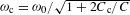and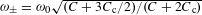, respectively, where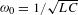is the static resonance frequency of each tank. Then, if the amplitude of the capacitance modulation is ΔCm, the frequency modulation amplitude is found as δωm = ω±ΔCm/(2C). The frequency ωc should be designed to be as far as possible from ω± in order for the common mode not to affect the operation of the structure at ω±, at which non-reciprocity occurs. In the lumped-element circuit of Fig. 2b this condition is satisfied by taking Cc, or equivalently by coupling the tanks through short circuits, yielding ωc = 0 and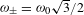.

The non-reciprocal response of the circuit of Fig. 2b is demonstrated by capacitively coupling it to three microstrip transmission lines, realizing a three-port device. Exciting the structure from, for example, port 1 at frequency ω± results in the excitation of |R〉 and |L〉 with the same amplitude and opposite phase φR = − φL, owing to the symmetrical distribution of these states around ω±. Then, the signals at ports 2 and 3 are proportional to $e i 2 π / 3 e i ϕ R +e i 4 π / 3 e i ϕ L$ and $e − i 2 π / 3 e i ϕ R +e − i 4 π / 3 e i ϕ L$, respectively, as the superposition of |R〉 and |L〉 at these ports. If δωm and ωm are selected so that φR = − φL = π/6, the signal at port 3 is identically zero, while the signal at port 2 is non-zero, routing the incident power from port 1 to port 2. Owing to the symmetry of the structure with respect to its ports, incident power from ports 2 and 3 is similarly routed to ports 3 and 1, thus realizing the functionality of a non-reciprocal circulator with infinite isolation. Notice that the above description assumes a weak excitation of the common state, which makes clear the importance of choosing its resonance frequency as far as possible from the resonance frequency of the rotating states.

The realized device was designed to resonate at 170 MHz with a Q-factor of about 10 for Vd.c. = 1.99 V and Vm = 0. The modulation frequency was set to 15 MHz, in order for the intermodulation by-products at frequencies ω ± ωm, created by the secondary substates of |R〉 and |L〉, to fall outside the resonance band, whose bandwidth is here around 10 MHz. The exact values of the circuit components and its full topology are provided in the Methods and Supplementary Methods. Figure 2c shows a photograph of the fabricated prototype. We underline here the deeply subwavelength size of the realized device (λ/75), simply based on three lumped resonant circuit tanks.

Without modulation, the signal is equally split at the two output ports, as expected from symmetry, and the system is fully reciprocal (Fig. 3a). When the modulation signal is switched on, the symmetry is broken and power is unequally split. By varying the modulation amplitude it is possible to find a value for which the signal entering port 1 is routed exclusively to port 2, corresponding to φR = − φL = π/6. This condition is satisfied for Vm = 0.6 V, as can be seen in Fig. 3b: at the resonance frequency of 170 MHz, power incident to ports 1, 2 and 3 is routed to ports 2, 3 and 1, respectively, demonstrating the operation of an ideal, magnetic-free, deeply subwavelength linear circulator. For comparison, Fig. 3c shows the S-parameters obtained using full-wave and circuit simulations: the agreement with the measurement is excellent.

To get a deeper insight into the effect of Vm on the device operation, Fig. 4a shows the transmission between ports 1 and 2 at resonance versus Vm. For Vm = 0, S21 = S12, as expected. Increasing Vm results in an increase of S21 and a decrease of S12 until Vm = 0.6 V, where S12 = 0. Past this point, S21 and S12 get closer, as expected when we depart from the destructive interference condition. For very large values of Vm, S21 and S12 both tend to zero, because the counter-rotating states move far from ω± and, therefore, are weakly excited at ω±. The magnitude of the asymmetry between S21 and S12 is measured by the isolation |S21/S12|, plotted in Fig. 4b on a logarithmic scale versus Vm. At the optimum modulation voltage Vm = 0.6 V, S21 is over four orders of magnitude larger than S12, indicating giant non-reciprocity, well above the levels of any commercial magnetic-based device.

Another unique property of the proposed device consists in its real-time tunability features. The biasing voltage Vd.c., which provides the reverse biasing condition for the varactor diodes, determines their static capacitance. Therefore, Vd.c. can be used to actively control the static resonance frequency of the LC tanks, and consequently the frequency band over which non-reciprocity occurs. Figure 4c shows the measured isolation versus frequency for Vd.c. varied between 1.73 V and 4.5 V. The non-reciprocal response of our device can be efficiently tuned between 150 MHz and 210 MHz, corresponding to a relative bandwidth of over 30%. Across all this range, our measured isolation is above 40 dB. This strong tuning capability is an additional advantage of the proposed device compared to conventional magnetic-based microwave circulators, and it may be exploited in scenarios requiring dynamic tuning to balance changes in temperature or in the environment. The electronic spin applied to the proposed coupled-resonator loop realizes the equivalent of a dynamically tunable, strongly biased ferromagnetic metamaterial substrate.

In addition to being an ideal replacement for microwave non-reciprocal components, with significant advantages in terms of size, integration, cost, linearity, tunability and noise reduction, our findings may become even more important when applied to different frequencies or other types of waves, such as light or sound. For instance, this concept may be disruptive for integrated nanophotonic technology, for which optical non-reciprocal components are critical for laser protection and signal routing. At visible frequencies, electro-optic modulation in silicon-based components is typically achieved by means of carrier injection/depletion24,25, which can provide relatively strong permittivity modulation, but is typically accompanied by significant loss and low modulation frequencies for large modulation amplitudes. These side effects impose limitations on the applicability of the principle of angular-momentum biasing in uniform micro-ring resonators2,3, as discussed before. In contrast, the concept presented here ensures maximum modulation efficiency, significantly relaxing the requirements in terms of modulation amplitude. This in turn allows large quality factors and large modulation frequencies, which translate into strong non-reciprocal response in deeply subwavelength devices. We also envisage the realization of the proposed rotating modulation of coupled-resonator loops in photonic crystal technology, for which high-Q-factor coupled cavities may be implemented and efficiently modulated26. Our study also represents a new demonstration of the exciting possibilities offered by dynamic modulation of coupled-resonator networks, with unique control over the flow of light, in the context of recently presented concepts of photonic topological edge states and effective magnetic fields for photons27,28,29.

## Methods

### Modes of the coupled-resonators loop.

The coupled-mode equations of the system of Fig. 1 read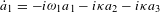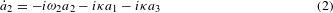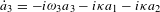where a1, a2 and a3 are the complex amplitudes of the three resonators. Equation (2) can be written in the more compact form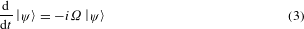where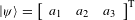is the state vector of the system and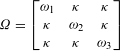In the absence of modulation, ω1, ω2 and ω3 are equal to ω0. Then, the eigenfrequencies and the corresponding state vectors of the system are found by the eigenvalues and eigenvectors of Ω, respectively. In particular, it can be shown that there are three states, a common state with resonant frequencyωc = ω0 + 2κ and state vector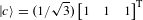, a right-handed rotating state with resonant frequency ω+ = ω0κ and state vector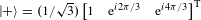, and a left-handed rotating state with resonant frequency ω = ω+ and state vector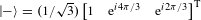. Note that because the eigenvalues ω+ and ω are degenerate, the eigenvectors |+〉 and |−〉 are not the only ones corresponding to these eigenvalues. As a matter of fact, any linear combination of |+〉 and |−〉, such as the vectors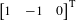and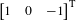, are also valid eigenvectors of ω+ and ω. However, hereafter, we will use |+〉 and |−〉, because they bear an immediate physical meaning as counter-rotating states of the coupled-resonators loop, and they significantly simplify the mathematical analysis.

In the presence of modulation ω1(t) = ω0 + δωm cos(ωmt), ω2(t) = ω0 + δωm cos(ωmt + 2π/3) and ω3(t) = ω0 + δωm cos(ωmt + 4π/3) result in a time-dependent Ω. In this case, the eigenstates of the system cannot be found by the eigenvalues and eigenvectors of Ω, and a full solution of equation (3) is necessary. Because modulation constitutes a perturbation of the coupled system, it is convenient to express the eigenstates of the modulated system in terms of the eigenstates of the coupled non-modulated system. To this end, equation (3) is expressed in the basis of |c〉, |+〉 and |−〉, by multiplying |ψ〉 with the conjugate transpose of the matrix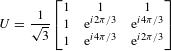with columns the state vectors |c〉, |+〉 and |−〉. In the new basis,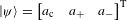, where ac, a+ and a are the complex amplitudes of the common, right- and left-handed states, respectively, and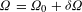whereIf ωm | ωcω±|, coupling between the common and rotating states, corresponding to the first row and column of δΩ, can be neglected and equation (3) simplifies to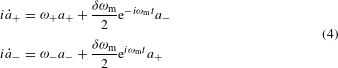It can be seen that equation (4) is satisfied if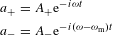where A+, A and ω are constants. Then, equation (4) becomes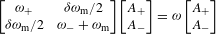which is an eigenvalue problem with respect to ω. The eigensolutions of this problem yield the eigenstates of the modulated system, as given by equation (1).

### Modulation amplitude of the coupled-resonators system.

We assume a resonator whose modal distribution fully resides in materials with permittivity ɛ, permeability μ, and resonant frequency ω0. It can be shown from perturbation theory that a small change Δɛ of the permittivity results in the following change of the resonance frequency30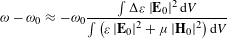where E0 and H0 are the resonant electric and magnetic field, respectively, and integration is performed all over the volume of the resonator. Furthermore, it is known that at resonance the electric and magnetic energies are equal, hence ∫ ɛ |E0| 2dV = ∫ μ |H0| 2dV and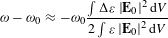If, in addition, ɛ and Δɛ are uniform over the volume where most of the resonator’s energy is concentrated, ∫ Δɛ |E0| 2dV Δɛ∫ |E0| 2dV and ∫ ɛ |E0| 2dV ɛ∫ |E0| 2dV, showing that the frequency modulation, δωm, for a permittivity modulation amplitude Δɛm is equal to δωm = ω0Δɛ/(2ɛ).

### Description of the experimental set-up.

The complete experimental set-up is shown in Supplementary Fig. 2 and a list of the associated equipment is provided in Supplementary Table 2. A waveform generator provides the modulation signal, which is split into three equal parts by means of a power divider. The output signals are then led to three phase shifters, which provide the required phase difference of 120° for the modulation signals of the three coupled resonators. The phase shifters are powered by a d.c. source and their phase shift is controlled by potentiometers. The outputs of the phase shifters are connected to the low-pass ports of three diplexers, whose outputs are connected to the RF/modulation ports of the ring. The high-pass ports of two of the diplexers are connected to the VNA ports while the high-pass port of the third diplexer is terminated to a matched load. The diplexers combine the modulation and RF signals and at the same time provide infinite isolation between the RF and modulation paths. By rotating the diplexers, which are connected to the VNA ports, it is possible to measure all the S-parameters of the circuit. The d.c. signal for biasing of the varactors is provided by a d.c. source connected to ports 4, 5 and 6 of the ring.

## References

1. 1

Fleury, R., Sounas, D. L., Sieck, C. F., Haberman, M. R. & Alù, A. Sound isolation and giant linear nonreciprocity in a compact acoustic circulator. Science 343, 516–519 (2014).

2. 2

Sounas, D. L., Caloz, C. & Alù, A. Giant non-reciprocity at the subwavelength scale using angular momentum-biased metamaterials. Nature Commun. 4, 2407 (2013).

3. 3

Sounas, D. L. & Alù, A. Angular-momentum-biased nanorings to realize magnetic-free integrated optical isolation. ACS Photon. 1, 198–204 (2014).

4. 4

Tanaka, S., Shimimura, N. & Ohtake, K. Active circulators—The realization of circulators using transistors. Proc. IEEE 53, 260–267 (1965).

5. 5

Hwang, I. K., Yun, S. H. & Kim, B. Y. All-fiber-optic nonreciprocal modulator. Opt. Lett. 22, 507–509 (1997).

6. 6

Bhandare, S. et al. Novel nonmagnetic 30-dB traveling-wave single-sideband optical isolator integrated in III/V material. IEEE J. Sel. Top. Quantum Electron. 11, 417–421 (2005).

7. 7

Galland, C., Ding, R., Harris, N. C., Baehr-Jones, T. & Hochberg, M. Broadband on-chip optical non-reciprocity using phase modulators. Opt. Express 21, 14500–14511 (2013).

8. 8

Doerr, C. R., Chen, L. & Vermeulen, D. Silicon photonics broadband modulation-based isolator. Opt. Express 22, 4493–4498 (2014).

9. 9

Kodera, T., Sounas, D. L. & Caloz, C. Artificial Faraday rotation using a ring metamaterial structure without static magnetic field. Appl. Phys. Lett. 99, 03114 (2011).

10. 10

Wang, Z. et al. Gyrotropic response in the absence of a bias field. Proc. Natl Acad. Sci. USA 109, 13194–13197 (2012).

11. 11

Soljačić, M., Luo, C., Joannopoulos, J. D. & Fan, S. Nonlinear photonic crystal microdevices for optical integration. Opt. Lett. 28, 637–639 (2003).

12. 12

Shadrivov, I. V., Fedotov, V. A., Powell, D. A., Kivshar, Y. S. & Zheludev, N. I. Electromagnetic wave analogue of an electronic diode. New J. Phys. 13, 033025 (2011).

13. 13

Fan, L. et al. An all-silicon passive optical diode. Science 335, 447–450 (2012).

14. 14

Peng, P. et al. Parity-time-symmetric whispering-gallery microcavities. Nature Phys. 10, 394–398 (2014).

15. 15

Yu, Z. & Fan, S. Complete optical isolation created by indirect interband photonic transitions. Nature Photon. 3, 91–94 (2009).

16. 16

Lira, H., Yu, Z., Fan, S. & Lipson, M. Electrically driven nonreciprocity induced by interband photonic transition on a silicon chip. Phys. Rev. Lett. 109, 033901 (2012).

17. 17

Huang, X. & Fan, S. Complete all-optical silica fiber isolator via stimulated Brillouin scattering. J. Lightwave Technol. 29, 2267–2274 (2011).

18. 18

Kang, M. S., Butsch, A. & Russell, P. St. J. Reconfigurable light-driven opto-acoustic isolators in photonic crystal fibre. Nature Photon. 5, 549–553 (2011).

19. 19

Fang, K., Yu, Z. & Fan, S. Photonic Aharonov–Bohm effect based on dynamic modulation. Phys. Rev. Lett. 108, 153901 (2012).

20. 20

Hafezi, M. & Rabl, P. Optomechanically induced non-reciprocity in microring resonators. Opt. Express 20, 7672–7684 (2012).

21. 21

Poulton, C. G. et al. Design for broadband on-chip isolator using stimulated Brillouin scattering in dispersion-engineered chalcogenide waveguides. Opt. Express 20, 21235–21246 (2012).

22. 22

Wang, D-W. et al. Optical diode made from a moving photonic crystal. Phys. Rev. Lett. 110, 093901 (2013).

23. 23

Kamal, A., Clarke, J. & Devoret, M. H. Noiseless non-reciprocity in a parametric active device. Nature Phys. 7, 311–315 (2011).

24. 24

Xu, Q., Schmidt, B., Pradhan, S. & Lipson, M. Micrometer-scale silicon electro-optic modulator. Nature 435, 325–327 (2005).

25. 25

Xu, Q., Manipatruni, S., Schmidt, B., Shakya, J. & Lispon, M. 12.5 Gbits/s carrier-injection-based silicon micro-ring silicon modulators. Opt. Express 15, 430–436 (2007).

26. 26

Shambat, G. et al. Ultra-low power fiber-coupled gallium arsenide photonic crystal cavity electro-optic modulator. Opt. Express 19, 7530–7536 (2011).

27. 27

Hafezi, M., Demler, E., Lukin, M. & Taylor, J. Robust optical delay lines with topological protection. Nature Phys. 7, 907–912 (2011).

28. 28

Hafezi, M., Mittal, S., Fan, J., Migdall, A. & Taylor, J. Imaging topological edge states in silicon photonics. Nature Photon. 7, 1001–1005 (2013).

29. 29

Fang, K., Yu, Z. & Fan, S. Realizing effective magnetic field for photons by controlling the phase of dynamic modulation. Nature Photon. 6, 782–787 (2012).

30. 30

Haus, H. A. Waves and Fields in Optoelectronics (Prentice Hall, 1984).

## Acknowledgements

The work was supported in part by AFOSR with YIP award No. FA9550-11-1-0009 and DTRA with YIP award No. HDTRA1-12-1-0022.

## Author information

Authors

### Contributions

N.A.E. performed the experiment. D.L.S. and N.A.E. designed the structure and conducted the numerical calculations and theoretical modelling. J.S. helped in the selection and modelling of the modulation varactors. A.A. directed and supervised the project. All authors have read and commented on the paper.

### Corresponding author

Correspondence to Andrea Alù.

## Ethics declarations

### Competing interests

The authors declare no competing financial interests.

## Supplementary information

### Supplementary Information

Supplementary Information (PDF 536 kb)

## Rights and permissions

Reprints and Permissions

• ### Phase-Induced Frequency Conversion and Doppler Effect With Time-Modulated Metasurfaces

• Davide Ramaccia
• , Dimitrios L. Sounas
• , Andrea Alu
• , Alessandro Toscano
•  & Filiberto Bilotti

IEEE Transactions on Antennas and Propagation (2020)

• ### Effective medium concept in temporal metamaterials

• Victor Pacheco-Peña

Nanophotonics (2020)

• ### Design and modulation of the plasmon-induced transparency based on terahertz metamaterials

• Min Zhong

Infrared Physics & Technology (2020)

• ### Serrodyne Frequency Translation Using Time-Modulated Metasurfaces

• Zhanni Wu
•  & Anthony Grbic

IEEE Transactions on Antennas and Propagation (2020)

• ### Linear isolators using wavelength conversion

• Kamal Abdelsalam
• , Tengfei Li
• , Jacob B. Khurgin
•  & Sasan Fathpour

Optica (2020)